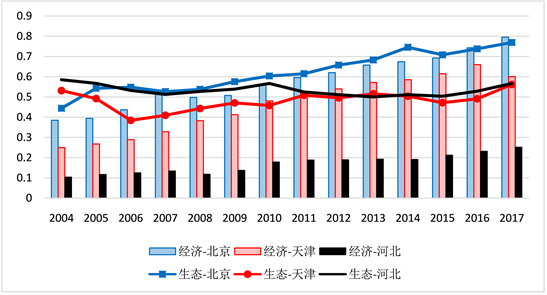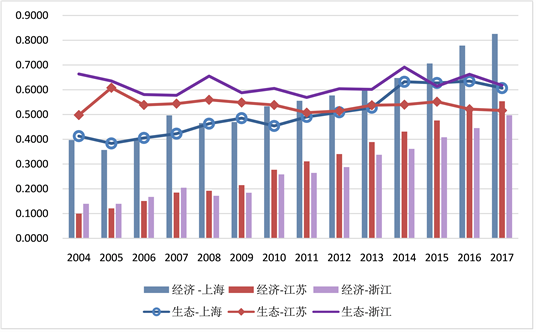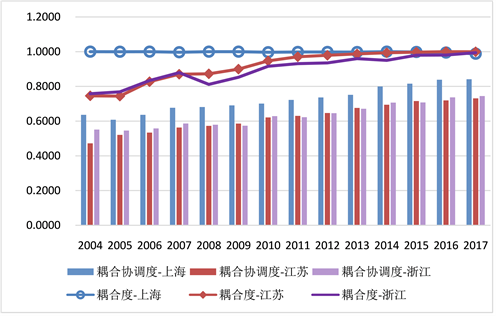﻿ 中国城市群生态与经济系统耦合协调发展研究

# 中国城市群生态与经济系统耦合协调发展研究Study on Coupling Coordinated Development of City-Group’s Ecosystem and Economic System in China

Abstract: Empirically analyze the cooperative development of Yangtze River Delta and Beijing-Tianjin-Hebei region’s ecological and economic system based on coupling coordination degree model from 2004 to 2017. The result shows high correlation between their ecological system and economic system of two city groups, but their coupling coordination degree is different. Beijing-Tianjin-Hebei region is relatively large disparity: Beijing is top-level coupling coordination degree, Tianjin is second- level and Hebei is economic-backward of second-level coupling coordination degree; The coordinated development of Yangtze River Delta is more balanced. Although only Shanghai has entered into coordinated development, there is little difference with Jiangsu and Zhejiang, and they are also about to enter the stage of coordinated development. Overall, coupling coordination degree of Yangtze River Delta is better than Beijing-Tianjin-Hebei region.

1. 引言

2021年4月30日下午，中共中央政治局就新形势下加强我国生态文明建设进行了第二十九次集体学习，习近平总书记再次强调，生态环境保护和经济发展是辩证统一、相辅相成的，建设生态文明、推动绿色低碳循环发展，不仅可以满足人民日益增长的优美生态环境需要，而且可以推动实现更高质量、更有效率、更加公平、更可持续、更为安全的发展。进入新发展阶段以来，中国城市群与区域一体化的发展正不断驱动着经济的高质量发展与跨越，而长三角与京津冀作为我国经济发展格局中最具活力、功能最全、综合价值最高的两大城市群，在实现区域一体化发展的过程中，必须统筹兼顾经济、生活、生态、安全等多元需求。因此，应积极推进城市群的生态系统与经济系统的协同发展，以充分发挥城市群对高质量发展的强大作用。而采用恰当的研究方法对京津冀与长三角城市群生态–经济系统的协同发展现状进行测度，并比较分析两大城市群的异同，找到影响我国城市群生态系统与经济系统协同发展的具体因素以制定有效应对策略予以改善提高，是今后五年乃至十五年内实现中国城市群高质量协同发展的重要途径。

2. 理论模型构建

2.1. 耦合协调度模型介绍

${w}_{ij}=\frac{{r}_{ij}^{+}}{{\sum }_{i=1}^{m}{r}_{ij}^{+}}$${w}_{ij}=\frac{{r}_{ij}^{-}}{{\sum }_{i=1}^{m}{r}_{ij}^{-}}$ (1)

${e}_{j}=-k\underset{i=1}{\overset{m}{\sum }}{w}_{ij}\mathrm{ln}{w}_{ij}$$k={\left(\mathrm{ln}m\right)}^{-1}$ (2)

${\phi }_{j}=1-{e}_{j}$ (3)

${w}_{j}=\frac{{\phi }_{j}}{{\sum }_{j=1}^{n}{\phi }_{j}}$ (4)

$f\left(G\right)=\sum {w}_{j}^{g}{r}_{ij}^{g}$ (5)

$f\left(E\right)=\sum {w}_{j}^{e}{r}_{ij}^{e}$ (6)

$C=\sqrt{\frac{f\left(G\right)×f\left(E\right)}{{\left[\alpha f\left(G\right)+\beta f\left(E\right)\right]}^{2}}}$ (7)

$D=\sqrt{C×T}$ (8)

$T=\alpha f\left(G\right)+\beta f\left(E\right)$ (9)Table 1. Classification of coupling and coordination types of ecosystems and economic systems

2.2. 现状描述Table 2. System indicators and their weights in Beijing-Tianjin-HebeiTable 3. The system indicators and their weights in the Yangtze River DeltaFigure 1. Evaluation of the development of the Beijing-Tianjin-Hebei ecosystem and economic systemFigure 2. Evaluation of the Yangtze River Delta ecosystem and economic system development

3. 现状评价

3.1. 耦合度评价Table 4. Evaluation of the eco-economic system coupling Degree of Beijing-Tianjin-Hebei urban agglomerationTable 5. Evaluation of the coupling degree of the ecological-economic system of the urban agglomeration in the Yangtze River Delta

3.2. 耦合协调度评价Table 6. Evaluation of the coupling coordination degree of the ecological-economic system of the Beijing-Tianjin-Hebei urban agglomerationFigure 3. Evaluation of the ecological-economic system coupling degree and coupling coordination degree of the Beijing-Tianjin-Hebei urban agglomerationTable 7. Evaluation of the coupling coordination degree of ecological-economic system in the Yangtze River Delta urban agglomerationFigure 4. Evaluation of the ecological-economic system coupling degree and coupling coordination degree of the urban agglomeration in the Yangtze River Delta

4. 小结

2021年度全国党校(行政学院)系统重点调研课题“新发展阶段生态系统与经济系统协同发展研究”(课题编号：2021DXXTZDDYKT007)的阶段性成果。

 Grossman, G.M. and Krueger, A.B. (1995) Economic growth and the environment. The Quarterly Journal of Econom-ics, 110, 353-377.
https://doi.org/10.2307/2118443

 Senbel, M., Mcdaniels, T. and Dowlatabadi, H. (2003) The Ecological Footprint: A Non-Monetary Metric of Human Consumption Applied to North America. Global Envi-ronmental Change, 13, 83-100.
https://doi.org/10.1016/S0959-3780(03)00009-8

 Kahuthu, A. (2006) Economic Growth and Environmental Degradation in a Global Context. Environment Development & Statistics, 79, 4.

 Galli, A., Kitzes, J. and Niccolucci, V. (2012) Assessing the Global Environmental Consequences of Economic Growth through the Ecological Footprint: A Focus on China and India. Ecological Indicators, 17, 99-107.
https://doi.org/10.1016/j.ecolind.2011.04.022

 范慧平, 孟天醒, 文倩, 宋文博. 河南省生态环境与经济增长关系的动态计量分析[J]. 河南农业大学学报, 2015, 49(4): 511-516+523.

 王勇, 李雅楠, 李建民. 环境规制、劳动力再配置及其宏观含义[J]. 经济评论, 2017(2): 33-47.

 吴玉鸣, 张燕. 中国区域经济增长与环境的耦合协调发展研究[J]. 资源科学, 2008, 30(1): 25-30.

 潘明明, 蔡玉婧, 蒋世辉. 区域经济、旅游、生态环境系统耦合协调发展研究[J]. 新疆农垦经济, 2014(1): 21-27.

 谢炳庚, 陈永林, 李晓青. 耦合协调模型在“美丽中国”建设评价中的运用[J]. 经济地理, 2016(7): 38-44.

 刘德光, 屈小爽. 中国旅游经济与生态环境协调发展度测算及区域差异分析[J]. 广东财经大学学报, 2016, 31(4): 89-96+105.

 邓淇中, 邹雨情. 农业生态环境与经济增长耦合协调度时空分异研究——以湖南省为例[J]. 湖南财政经济学院学报, 2018, 34(1): 37-44.

 马双, 张翼鸥. 长三角城市群生态环境-科技创新-经济增长耦合协调时空分异研究[J]. 上海经济, 2019(5): 23-32.

 王芳. 基于耦合协调度模型的生态系统与经济系统协同发展研究——以京津冀地区为例[J]. 湖北社会科学, 2021(6): 64-72.

Top